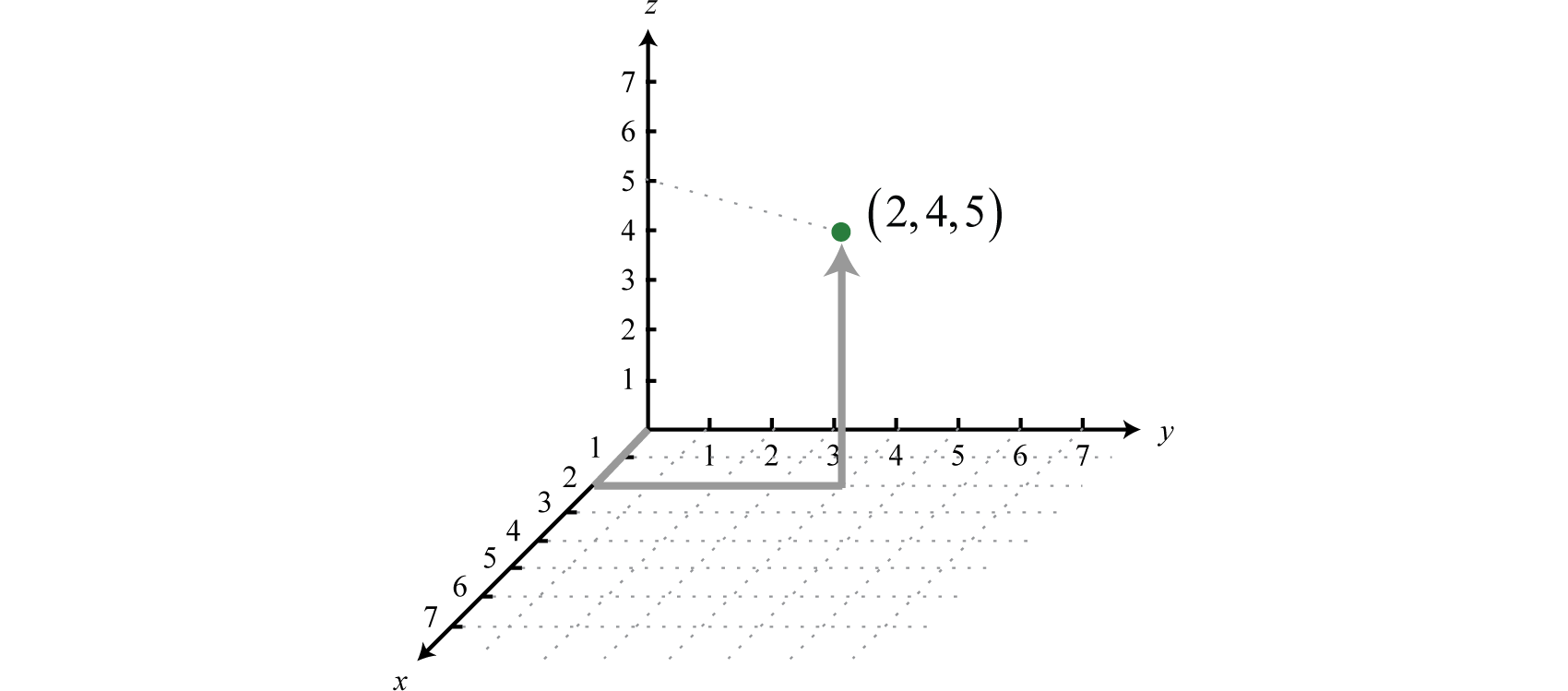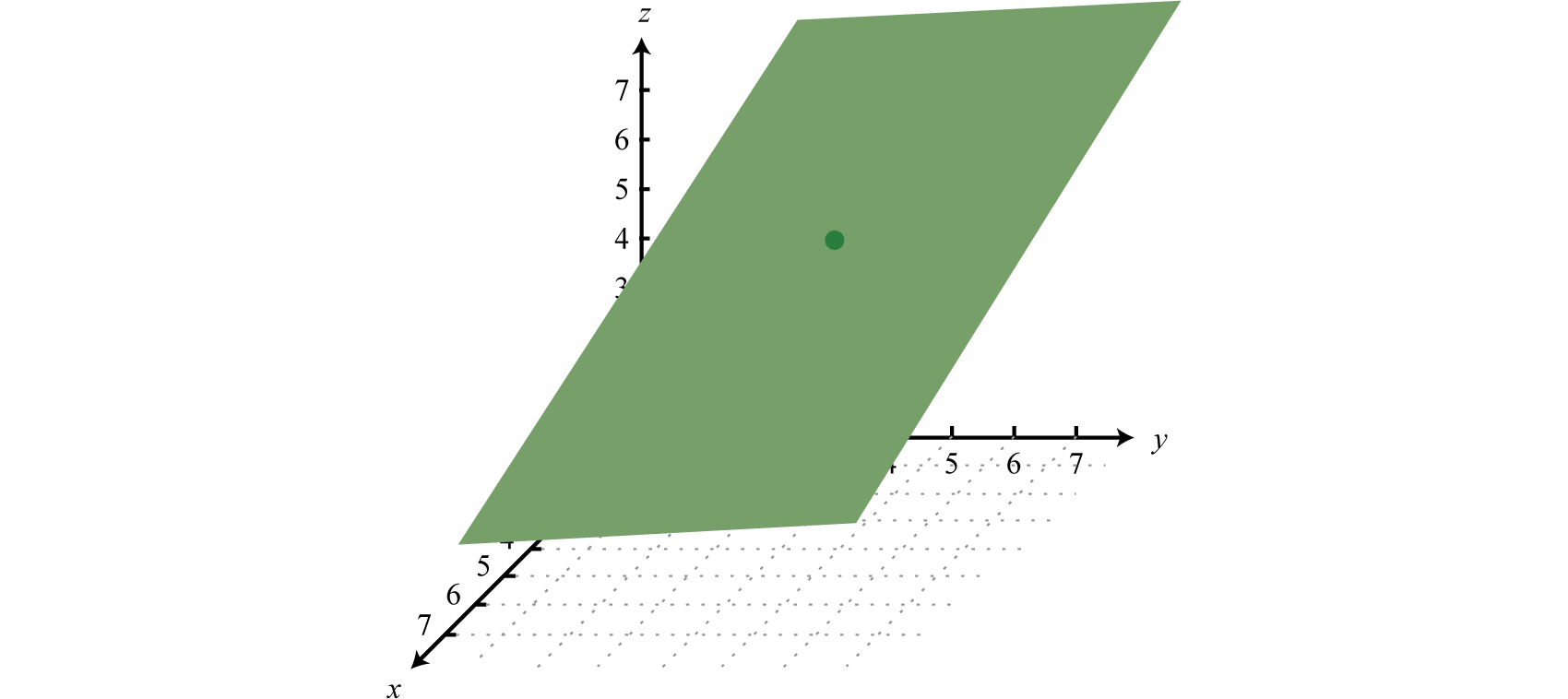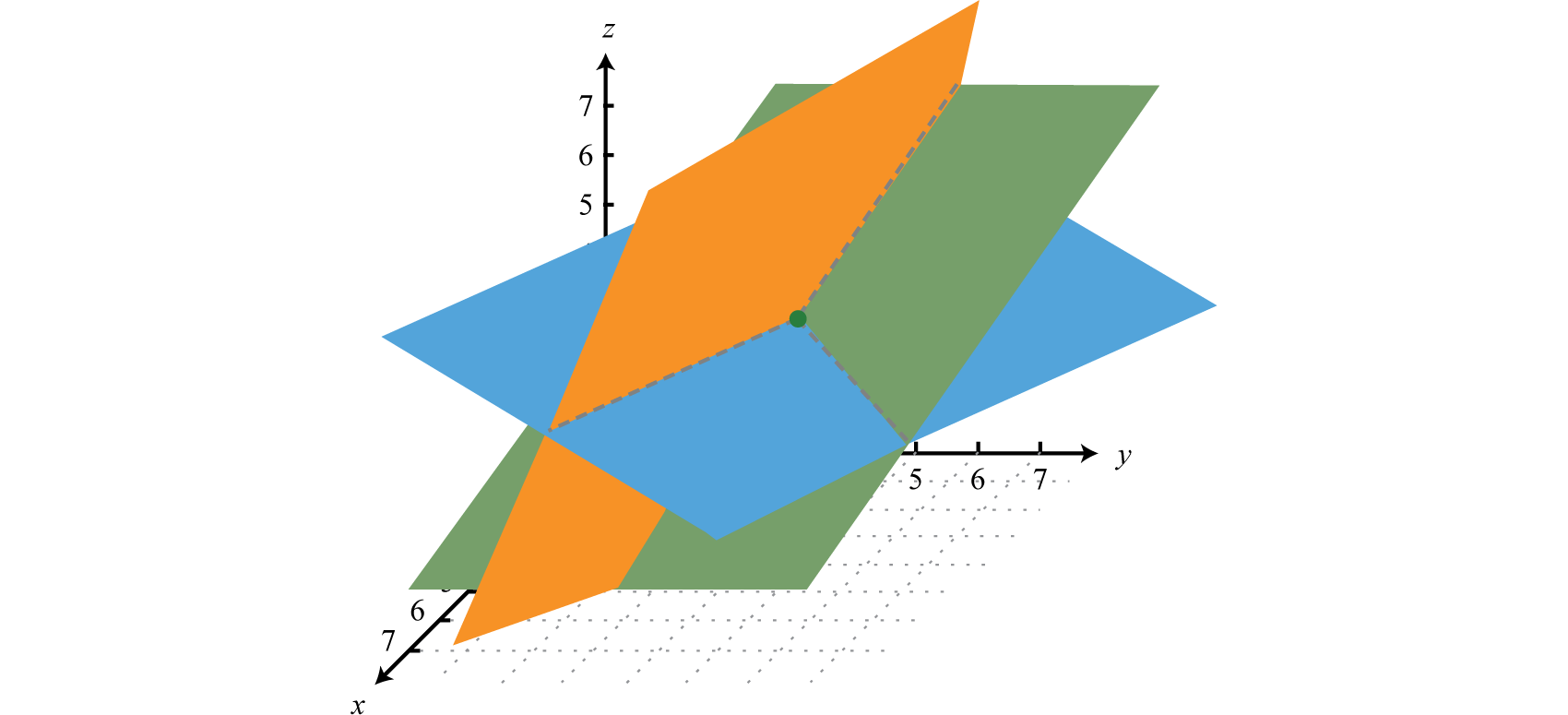This is “Solving Linear Systems with Three Variables”, section 3.4 from the book Advanced Algebra (v. 1.0). For details on it (including licensing), click here.

Has this book helped you? Consider passing it on:
Creative Commons supports free culture from music to education. Their licenses helped make this book available to you.
DonorsChoose.org helps people like you help teachers fund their classroom projects, from art supplies to books to calculators.

## 3.4 Solving Linear Systems with Three Variables

### Learning Objectives

1. Check solutions to linear systems with three variables.
2. Solve linear systems with three variables by elimination.
3. Identify dependent and inconsistent systems.
4. Solve applications involving three unknowns.

## Solutions to Linear Systems with Three Variables

Real-world applications are often modeled using more than one variable and more than one equation. In this section, we will study linear systems consisting of three linear equations each with three variables. For example,

${3x+2y− z=−7(1)6x− y+3z=−4(2)x+10y−2z=2(3)$

A solution to such a linear system is an ordered tripleTriples (x, y, z) that identify position relative to the origin in three-dimensional space. (x, y, z) that solves all of the equations. In this case, (−2, 1, 3) is the only solution. To check that an ordered triple is a solution, substitute in the corresponding x-, y-, and z-values and then simplify to see if you obtain a true statement from all three equations.

$Check: (−2,1,3)$

$Equation (1):3x+2y+z=−73(−2)+2(1)−(3)=−7−6+2−3=−7−7=−7 ✓$

$Equation (2):6x−y+3z=−46(−2)−(1)−3(3)=−4−12−1−9=−4−4=−4 ✓$

$Equation (3):x+10y−2z=2(−2)+10(1)−2(3)=2−2+10−6=22=2 ✓$

Because the ordered triple satisfies all three equations we conclude that it is indeed a solution.

### Example 1

Determine whether or not $(1,4,43)$ is a solution to the following linear system:

${9x+y−6z=5−6x−3y+3z=−143x+2y−7z=15 .$

Solution:

$Check: (1,4,43)$

$Equation (1):9x+y−6z=59(1)+(4)−6(43)=59+4−8=55=5 ✓$

$Equation (2):−6x−3y+3z=−146(1)−3(4)+3(43)=14−6−12+4=−14−14=−14 ✓$

$Equation (3):3x+2y−7z=153(1)+2(4)−7(43)=153+8−283=1553=15 ✗$

Answer: The point does not satisfy all of the equations and thus is not a solution.

An ordered triple such as $(2,4,5)$ can be graphed in three-dimensional space as follows:The ordered triple indicates position relative to the origin (0, 0, 0), in this case, 2 units along the x-axis, 4 units parallel to the y-axis, and 5 units parallel to the z-axis. A linear equation with three variablesAn equation that can be written in the standard form $ax+by+cz=d$ where a, b, c, and d are real numbers. is in standard form if $ax+by+cz=d$ where a, b, c, and d are real numbers. For example, $6x+y+2z=26$ is in standard form. Solving for z, we obtain $z=−3x−12y+13$ and can consider both x and y to be the independent variables. When graphed in three-dimensional space, its graph will form a straight flat surface called a planeAny flat two-dimensional surface..Therefore, the graph of a system of three linear equations and three unknowns will consist of three planes in space. If there is a simultaneous solution, the system is consistent and the solution corresponds to a point where the three planes intersect.Graphing planes in three-dimensional space is not within the scope of this textbook. However, it is always important to understand the geometric interpretation.

Try this! Determine whether or not (3, −1, 2) a solution to the system: ${ 2x−3y−z=73x+5y−3z=−24x−y+2z=17$.

Answer: Yes, it is a solution.

## Solve Linear Systems with Three Variables by Elimination

In this section, the elimination method is used to solve systems of three linear equations with three variables. The idea is to eliminate one of the variables and resolve the original system into a system of two linear equations, after which we can then solve as usual. The steps are outlined in the following example.

### Example 2

Solve: ${3x+2y−z=−7 (1)6x−y+3z=−4 (2) .x+10y−2z=2 (3)$

Solution:

All three equations are in standard form. If this were not the case, it would be a best practice to rewrite the equations in standard form before beginning this process.

Step 1: Choose any two of the equations and eliminate a variable. In this case, we can line up the variable z to eliminate if we group 3 times the first equation with the second equation.$9x+6y −3z=21+6x−y + 3z=−415x+5y=−25✓$

Step 2: Choose any other two equations and eliminate the same variable. We can line up z to eliminate again if we group −2 times the first equation with the third equation.$−6x−4y + 2z=14+x+10y−2z=2−5x+6y=16✓$

Step 3: Solve the resulting system of two equations with two unknowns. Here we solve by elimination. Multiply the second equation by 3 to line up the variable x to eliminate.$15x+5y=−25+−15x+18y=4823y=23y=1$

Step 4: Back substitute and determine all of the coordinates. To find x use the following,

$15x+5y=−2515x+5(1)=−2515x=−30x=−2$

Now choose one of the original equations to find z,

$3x+2y−z=−7 (1)3(−2)+2(1)−z=−7−6+2−z=−7−4−z=−7−z=−3z=3$

Hence the solution, presented as an ordered triple (x, y, z), is (−2, 1, 3). This is the same system that we checked in the beginning of this section.

It does not matter which variable we initially choose to eliminate, as long as we eliminate it twice with two different sets of equations.

### Example 3

Solve: ${−6x−3y+3z=−149x+y−6z=53x+2y−7z=15$.

Solution:

Because y has coefficient 1 in the second equation, choose to eliminate this variable. Use equations 1 and 2 to eliminate y.Next use equations 2 and 3 to eliminate y again.This leaves a system of two equations with two variables x and z,

${21x−15z=1−15x+5z=5$

Multiply the second equation by 3 and eliminate the variable z.Now back substitute to find z.

$21x−15z=121(−23)−15z=1−14−15z=1−15z=15z=−1$

Finally, choose one of the original equations to find y.

$−6x−3y+3z=−14−6(−23)−3y+3(−1)=−144−3y−3=−141−3y=−14−3y=−15y=5$

Answer: $(−23,5,−1)$

### Example 4

Solve: ${2x+6y+7z=4−3x−4y+5z=125x+10y−3z=−13.$

Solution:

In this example, there is no obvious choice of variable to eliminate. We choose to eliminate x.

$(1)(2){2x+6y+7z=4−3x−4y+5z=12⇒×3⇒×2{6x+18y+21z=12−6x−8y+10z=24–10y+31z=36✓$

Next use equations 2 and 3 to eliminate x again.

$(2)(3){−3x−4y+5z=125x+10y−3z=−13⇒×5⇒×3{−15x−20y+25z=6015x+30y−9z=−39–10y+16z=21✓$

This leaves a system of two equations with two variables y and z,

${10y+31z=3610y+16z=21$

Multiply the first equation by −1 as a means to eliminate the variable y.Now back substitute to find y.

$10y+31z=3610y+31(1)=3610y+31=3610y=5y=510y=12$

Choose any one of the original equations to find x.

$2x+6y+7z=42x+6(12)+7(1)=42x+3+7=42x+10=42x=−6x=−3$

Answer: $(−3,12,1)$

Try this! Solve: ${ 2x−3y−z=73x+5y−3z=−24x−y+2z=17$.

## Dependent and Inconsistent Systems

Just as with linear systems with two variables, not all linear systems with three variables have a single solution. Sometimes there are no simultaneous solutions.

### Example 5

Solve the system: ${4x−y+3z=521x−4y+18z=7−9x+y−9z=−8$.

Solution:

In this case we choose to eliminate the variable y.

$(1)(3){4x−y+3z=5 −9x+y−9z=−8–−5x−6z=−3✓$

Next use equations 2 and 3 to eliminate y again.This leaves a system of two equations with two variables x and z,

${−5x− 6z=−3−15x−18z=−25$

Multiply the first equation by −3 and eliminate the variable z.Adding the resulting equations together leads to a false statement, which indicates that the system is inconsistent. There is no simultaneous solution.

A system with no solutions is an inconsistent system. Given three planes, no simultaneous solution can occur in a number of ways.Just as with linear systems with two variables, some linear systems with three variables have infinitely many solutions. Such systems are called dependent systems.

### Example 6

Solve the system: ${7x−4y+z=−153x+2y−z=−55x+12y−5z=−5$.

Solution:

Eliminate z by adding the first and second equations together.

$(1) (2){7x−4y+z=−153x+2y−z=−5– 10x−2y=−20 ✓$

Next use equations 1 and 3 to eliminate z again.This leaves a system of two equations with two variables x and y,

${10x−2y=−2040x−8y=−80$

Line up the variable y to eliminate by dividing the first equation by 2 and the second equation by −8.

${10x−2y=−2040x−8y=−80⇒÷2⇒÷(−8) {5x−y=−10−5x+y=10–0=0 True$

A true statement indicates that the system is dependent. To express the infinite number of solutions $(x,y,z)$ in terms of one variable, we solve for y and z both in terms of x.

$10x−2y=−20−2y=−10x−20−2y−2=−10x−20−2y=5x+10$

Once we have y in terms of x, we can solve for z in terms of x by back substituting into one of the original equations.

$7x−4y+z=−157x−4(5x+10)+z=−157x−20x−40+z=−15−13x−40+z=−15z=13x+25$

Answer: $(x,5x+10,13x+25)$.

A consistent system with infinitely many solutions is a dependent system. Given three planes, infinitely many simultaneous solutions can occur in a number of ways.Try this! Solve: ${7x+y−2z=−4−21x−7y+8z=47x+3y−3z=0$.

Answer: $(x,73x+4,143x+4)$

## Applications Involving Three Unknowns

Many real-world applications involve more than two unknowns. When an application requires three variables, we look for relationships between the variables that allow us to write three equations.

### Example 7

A community theater sold 63 tickets to the afternoon performance for a total of $444. An adult ticket cost$8, a child ticket cost $4, and a senior ticket cost$6. If twice as many tickets were sold to adults as to children and seniors combined, how many of each ticket were sold?

Solution:

Begin by identifying three variables.

Let x represent the number of adult tickets sold.

Let y represent the number of child tickets sold.

Let z represent the number of senior tickets sold.

The first equation comes from the statement that 63 tickets were sold.

$(1) x+y+z=63$

The second equation comes from total ticket sales.

$(2) 8x+4y+6z=444$

The third equation comes from the statement that twice as many adult tickets were sold as child and senior tickets combined.

$x=2(y+z)x=2y+2z(3) x−2y−2z=0$

Therefore, the problem is modeled by the following linear system.

${x+y+z=638x+4y+6z=444x−2y−2z=0$

Solving this system is left as an exercise. The solution is $(42,9,12)$.

Answer: The theater sold 42 adult tickets, 9 child tickets, and 12 senior tickets.

### Key Takeaways

• A simultaneous solution to a linear system with three equations and three variables is an ordered triple (x, y, z) that satisfies all of the equations. If it does not solve each equation, then it is not a solution.
• We can solve systems of three linear equations with three unknowns by elimination. Choose any two of the equations and eliminate a variable. Next choose any other two equations and eliminate the same variable. This will result in a system of two equations with two variables that can be solved by any method learned previously.
• If the process of solving a system leads to a false statement, then the system is inconsistent and has no solution.
• If the process of solving a system leads to a true statement, then the system is dependent and has infinitely many solutions.
• To solve applications that require three variables, look for relationships between the variables that allow you to write three linear equations.

### Part A: Linear Systems with Three Variables

Determine whether or not the given ordered triple is a solution to the given system.

1. $(3,−2,−1)$;

${x+y−z=22x−3y+2z=10x+2y+z=−3$
2. $(−8,−1,5)$;

${x+2y−z=−152x−6y+2z=03x−9y+4z=5$
3. $(1,−9,2)$;

${8x+y−z=−37x−2y−3z=19x−y+9z=28$
4. $(−4,1,−3)$;

${3x+2y−z=−7x−5y+2z=32x+y+3z=−16$
5. $(6,23,−12)$;

${x+6y−4z=12−x+3y−2z=−3x−9y+8z=−4$
6. $(14,−1,−34)$;

${2x−y−2z=34x+5y−8z=2x−2y−z=3$
7. $(3,−2,1)$;

${4x−5y=222y−z=8−5x+2z=−13$
8. $(1,52,−12)$;

${2y−6z=83x−4z=518z=−9$
9. $(12,−2,6)$;

${a−b+c=94a−2b+c=142a+b+12c=3$
10. $(−1,5,−7)$;

${3a+b+13c=−138a+2b+12c=−3225a+5b+c=−7$

### Part B: Solving Linear Systems with Three Variables

Solve.

1. ${2x−3y+z=45x+2y+2z=2x+4y−3z=7$
2. ${5x−2y+z=−92x+y−3z=−57x+3y+2z=6$
3. ${x+5y−2z=153x−7y+4z=−72x+4y−3z=21$
4. ${x−4y+2z=32x+3y−3z=93x+2y+4z=−1$
5. ${5x+4y−2z=−54x−y+3z=146x+3y−5z=−12$
6. ${2x+3y−2z=−43x+5y+3z=172x+y−4z=−8$
7. ${x+y−4z=19x−3y+6z=2−6x+2y−4z=−2$
8. ${5x−8y+z=5−3x+5y−z=−3−11x+18y−3z=−5$
9. ${x−y+2z=32x−y+3z=2−x−3y+4z=1$
10. ${x+y+z=8x−y+4z=−7−x−y+2z=1$
11. ${4x−y+2z=36x+3y−4z=−13x−2y+3z=4$
12. ${x−4y+6z=−13x+8y−2z=25x+2y−3z=−5$
13. ${3x−4y−z=75x−8y+3z=112x+6y+z=9$
14. ${3x+y−4z=66x−5y+3z=19x+3y−4z=10$
15. ${7x−6y+z=8−x+2y−z=4x+2y−2z=14$
16. ${−9x+3y+z=312x−4y−z=2−6x+2y+z=8$
17. ${a−b+c=94a−2b+c=142a+b+12c=3$
18. ${3a+b+13c=−138a+2b+12c=−3225a+5b+c=−7$
19. ${3x−5y−4z=−54x−6y+3z=−226x+8y−5z=20$
20. ${7x+4y−2z=82x+2y+3z=−43x−6y−7z=8$
21. ${9x+7y+4z=84x−5y−6z=−11−5x+2y+3z=4$
22. ${3x+7y+2z=−75x+4y+3z=52x−3y+5z=−4$
23. ${4x−3y=12y−3z=23x+2z=3$
24. ${5y−3z=−283x+2y=84y−7z=−27$
25. ${2x+3y+z=16y+z=42z=−4$
26. ${x−3y−2z=52y+6z=−14z=−6$
27. ${2x=106x−5y=303x−4y−2z=3$
28. ${2x+7z=2−4y=68y+3z=0$
29. ${5x+7y+2z=412x+16y+4z=1510x+13y+3z=14$
30. ${8x+12y−8z=52x+3y−2z=24x−2y+5z=−1$
31. ${17x−4y−3z=−25x+12y−2z=−922x+5y−4z=−13$
32. ${3x−5y−12z=72x−y−12z=−123x−8y+z=11$
33. ${4a−2b+3c=93a+3b−5c=−610a−6b+5c=13$
34. ${6a−2b+5c=−24a+3b−3c=−13a+5b+6c=24$

### Part C: Applications

Set up a system of equations and use it to solve the following.

1. The sum of three integers is 38. Two less than 4 times the smaller integer is equal to the sum of the others. The sum of the smaller and larger integer is equal to 2 more than twice that of the other. Find the integers.

2. The sum of three integers is 40. Three times the smaller integer is equal to the sum of the others. Twice the larger is equal to 8 more than the sum of the others. Find the integers.

3. The sum of the angles A, B, and C of a triangle is 180°. The larger angle C is equal to twice the sum of the other two. Four times the smallest angle A is equal to the difference of angle C and B. Find the angles.

4. The sum of the angles A, B, and C of a triangle is 180°. Angle C is equal to the sum of the other two angles. Five times angle A is equal to the sum of angle C and B. Find the angles.

5. A total of $12,000 was invested in three interest earning accounts. The interest rates were 2%, 4%, and 5%. If the total simple interest for one year was$400 and the amount invested at 2% was equal to the sum of the amounts in the other two accounts, then how much was invested in each account?

6. Joe invested his $6,000 bonus in three accounts earning $412$% interest. He invested twice as much in the account earning $412$% as he did in the other two accounts combined. If the total simple interest for the year was$234, how much did Joe invest in each account?

7. A jar contains nickels, dimes, and quarters. There are 105 coins with a total value of $8.40. If there are 3 more than twice as many dimes as quarters, find how many of each coin are in the jar. 8. A billfold holds one-dollar, five-dollar, and ten-dollar bills and has a value of$210. There are 50 bills total where the number of one-dollar bills is one less than twice the number of five-dollar bills. How many of each bill are there?

9. A nurse wishes to prepare a 15-ounce topical antiseptic solution containing 3% hydrogen peroxide. To obtain this mixture, purified water is to be added to the existing 1.5% and 10% hydrogen peroxide products. If only 3 ounces of the 10% hydrogen peroxide solution is available, how much of the 1.5% hydrogen peroxide solution and water is needed?

10. A chemist needs to produce a 32-ounce solution consisting of $834$% acid. He has three concentrates with 5%, 10%, and 40% acid. If he is to use twice as much of the 5% acid solution as the 10% solution, then how many ounces of the 40% solution will he need?

11. A community theater sold 128 tickets to the evening performance for a total of $1,132. An adult ticket cost$10, a child ticket cost $5, and a senior ticket cost$6. If three times as many tickets were sold to adults as to children and seniors combined, how many of each ticket were sold?

12. James sold 82 items at the swap meet for a total of $504. He sold packages of socks for$6, printed t-shirts for $12, and hats for$5. If he sold 5 times as many hats as he did t-shirts, how many of each item did he sell?

13. A parabola passes through three points $(−1,7)$, $(1,−1)$ and $(2,−2)$. Use these points and $y=ax2+bx+c$ to construct a system of three linear equations in terms of a, b, and c and then solve the system.

14. A parabola passes through three points $(−2,11)$, $(−1,4)$ and $(1,2)$. Use these points and $y=ax2+bx+c$ to construct a system of three linear equations in terms of a, b, and c and solve it.

### Part D: Discussion Board

1. On a note card, write down the steps for solving a system of three linear equations with three variables using elimination. Use your notes to explain to a friend how to solve one of the exercises in this section.

2. Research and discuss curve fitting. Why is curve fitting an important topic?

1. No

2. Yes

3. Yes

4. No

5. No

1. (2, −1, −3)

2. (4, 1, −3)

3. (1, −1, 3)

4. Ø

5. (5, −10, −6)

6. $(12,−2,−12)$
7. $(3, 12, 0)$
8. $(x,32x−3,2x−10)$
9. (1, −2, 6)

10. (−1, 2, −2)

11. (1,−3, 5)

12. (1, 1, 0)

13. (0, 1, −2)

14. (5, 0, 6)

15. $Ø$

16. $(x,2x−1,3x+2)$

17. (1, 2, 3)

1. 8, 12, 18

2. A = 20°, B = 40°, and C = 120°

3. The amount invested at 2% was $6,000, the amount invested at 4% was$2,000, and the amount invested at 5% was \$4,000.

4. 72 nickels, 23 dimes, and 10 quarters

5. 10 ounces of the 1.5% hydrogen peroxide solution and 2 ounces of water

6. 96 adult tickets, 20 child tickets, and 12 senior tickets were sold.

7. $a=1$, $b=−4$, and $c=2$